# GSEB Solutions Class 12 Maths Chapter 13 Probability Ex 13.1

Gujarat Board GSEB Textbook Solutions Class 12 Maths Chapter 13 Probability Ex 13.1 Textbook Questions and Answers.

## Gujarat Board Textbook Solutions Class 12 Maths Chapter 13 Probability Ex 13.1

Question 1.
Given that E and F are events such that P(E) = 0.6, P(F) = 0.3 and P(E ∩ F) = 0.2, find P(E/F) and P(F/E).
Solution:
(i) P(E/F) = $$\frac{E∩F}{P(F)}$$ = $$\frac{0.2}{0.3}$$ = $$\frac{2}{3}$$.
(ii) P(F/E) = $$\frac{P(E∩F)}{P(E)}$$ = $$\frac{0.2}{0.6}$$ = $$\frac{2}{6}$$ = $$\frac{1}{3}$$.Question 2.
Compute P(A/B), if P(B) = 0.5 and P(A ∩ B) = 0.32.
Solution:
P(A/B) = $$\frac{P(A∩B)}{P(B)}$$ = $$\frac{0.32}{0.5}$$ = $$\frac{32}{50}$$ = $$\frac{16}{25}$$.

Question 3.
If P(A) = 0.8, P(B) = 0.5 and P(B/A) = 0.4, find
(i) P(A ∩ B)
(ii) P(A/B)
(iii) P(A ∪ B)
Solution:
(i) P(B/A) = $$\frac{P(A∩B)}{P(B)}$$ ⇒ 0.4 = $$\frac{P(A∩B)}{0.8}$$
∴ P(A ∩ B) = 0.4 × 0.8 = 0.32.

(ii) P(A/B) = $$\frac{P(A∩B)}{P(B)}$$ = $$\frac{0.32}{0.5}$$ = $$\frac{32}{50}$$ = $$\frac{16}{25}$$.

(iii) P(A ∪ B) = P(A) + P(B) – P(A ∩ B)
= 0.8 + 0.5 – 0.32 = 1.30 – 0.32
= 0.98.

Question 4.
Evaluate P(A ∪ B), if 2P(A) = P(B) = $$\frac{5}{13}$$ and P(A/B) = $$\frac{2}{5}$$.
Solution:
We have:
2P(A) = P(B) = $$\frac{5}{13}$$
⇒ P(A) = $$\frac{5}{26}$$, P(B) = $$\frac{5}{13}$$.
So, P(A/B) = $$\frac{P(A∩B)}{P(B)}$$
or $$\frac{2}{5}$$ = $$\frac{P(A \cap B)}{\frac{5}{13}}$$
⇒ P(A ∩ B) = $$\frac{2}{5}$$ × $$\frac{5}{13}$$ = $$\frac{2}{13}$$.
∴ P(A ∪ B) = P(A) + P(B) – P(A ∩ B)
= $$\frac{5}{26}$$ + $$\frac{5}{13}$$ – $$\frac{2}{13}$$ = $$\frac{5+10-4}{26}$$ = $$\frac{11}{26}$$.Question 5.
If P(A) = $$\frac{6}{11}$$, P(B) = $$\frac{5}{11}$$ and P(A ∪B) = $$\frac{7}{11}$$, find
(i) P(A ∩ B)
(ii) P(A/B)
(iii) P(B/A)
Solution:
P(A ∪ B) = P(A) + P(B) – P(A ∩ B)
(i) P(A ∩ B) = P(A) + P(B) – P(A ∪ B)
= $$\frac{6}{11}$$ + $$\frac{5}{11}$$ – $$\frac{7}{11}$$ = $$\frac{4}{11}$$.

(ii)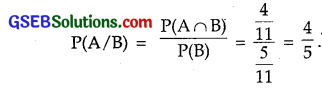(iii)Determine P(E/F) in the questions 6 to 9:

Question 6.
A coin is tossed three times, where
(i) E : head on third toss , F : Heads on first two tosses
(ii) E : at least two heads, F : at most two heads
(iii) E : at most two tails F : at least one tail.
Solution:
(i) E = Head occurs on third toss as
{HHH, HTH, THH, TTH}

F : Heads on first two tosses = {HHH, HHT}
∴ E ∩ F = {HHH}(ii) E : At least two heads = {HHT, HTH, THH, HHH}
F : At most two heads = {TTT, HTT, THT, HTT, HHT, HTH, THH}
∴ E ∩ F : {HHT, HTH, THH}(iii) E : At most two tails = {HHT, THT, TTH, HHT, HTH, THH, HHH}
F : At least one tail = {THH, HTH, HHT, TTH, THT, HTT, TTT}
∴ E ∩ F : {HTT, THT, TTH, THH, HTH, HHT}Question 7.
Two coins are tossed once, where
(i) E : tail appear on one coin ,F : one coin shows head
(ii) E : no tail appears, F : no head appears.
Solution:
(i) E : Tail appears on one coin = {TH, HT}
F : One coin shows head = {HT, TH}
∴ E ∩ F : {TH, HT}(ii) E : no tail appears = {HH}
F : no head appears = {TT}
So, E ∩ F = ∅
∴ P(E/F) = $$\frac{P(E∩F)}{P(F)}$$ = 0.

Question 8.
A dice is thrown three times, where
E : 4 appears on the third toss, F : 6 and 5 appears respectively on first two tosses.
Solution:
A dice is thrown three times.
E : 4 appears on third less
= {(1, 1, 4), (1, 2, 4), (1, 3, 4) (1, 6, 4)
(2, 1, 4, (2, 2, 4), (2, 3, 4) ……………… (2, 6, 4)
(3, 1, 4), (3, 2, 4), (3, 3, 4) …………………. (3, 6, 4)
(4, 1, 4), (4, 2, 4), (4, 3, 4) ………………….. (4, 6, 4)
(5, 1, 4), (5, 2, 4), (5, 3, 4) …………………. (5, 6, 4)
(6, 1, 4), (6, 2, 4), (6, 3, 4) …………………… (6, 6, 4)}
These are 36 cases.
F : 6 and 5 appears respectively on first two tosses
= {(6, 5, 1, (6, 5, 2), (6, 5, 3), (6, 5, 4), (6, 5, 5), (6, 5, 6)}
These are six cases.
Here, E ∩ F = {6, 5, 4}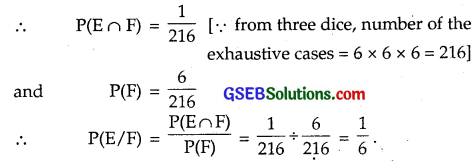Question 9.
Mother, father and son line up at random for a family picture, such that:
E : Son on one end
F : Father in middle
Solution:
Mother (m), Father (f) and Son (s) line up at random.
E : Son on one end : {(s, m, f), (s, f, m), (f, m, s), (m, f, s)}
F : Father in middle : {(m, f, s), (s, f, m)}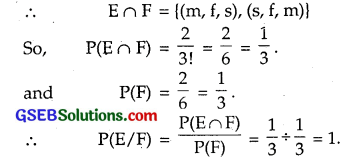Question 10.
A black and red dice are rolled:
(a) Find the conditional probability of obtaining a sum greater than 9, given that the black dice resulted in a 5.
(b) Find the conditional probability of obtaining the sum of 8, given that the red dice resulted in a number less than 4.
Solution:
(a) When two dice are thrown, a sum greater than 9 may be obtained as
A : {4, 6), (5, 5), (6, 4), (5, 6), (6, 5), (6, 6)}
B : Black dice will result in 5.
⇒ 5 is on black dice, any other number on red dice, i.e.,
= {(5, 1), (5, 2), (5, 3), (5, 4), (5, 5), (5, 6)}
So, A ∩ B = {(5, 5), (5, 6)}(b) A : Sum 8 is obtained = {(2, 6), (3, 5), (4, 4), (5, 3), (6, 2)}
B : red dice results in a number less than 4.
Either first or second dice is red
B = {(1, 1), (1, 2), (1, 3), (1, 4), (1, 5), (1, 6),
(2, 1), (2, 2), (2, 3), (2, 4), (2, 5), (2, 6),
(3, 1), (3, 2), (3, 3), (3, 4), (3, 5), (3, 6)}
So, A ∩ B = {(2, 6), (3, 5)}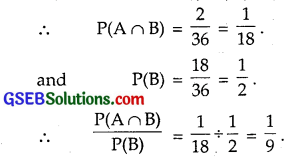Question 11.
A fair dice is rolled. Consider the event E = {1, 3, 5} F = {2, 3} and G = {2, 3, 4, 5}. Find
(i) P(E/F) and P(F/E)
(ii) P(E/G), P(G/E)
(iii) P(E ∪ F/G) and P(E ∩ F/G)
Solution:
(i) E = {1, 3, 5}, F = {2, 3}, E ∩ F = {3}.(ii) E = {1, 3, 5}, G = {2, 3, 4, 5}, E ∩ G = {3, 5}.(iii) E = {1, 3, 5}, F = {2, 3}, G = {2, 3, 4, 5}
E ∩ G = {3, 5}, F ∩ G = {2, 3}, (E ∩ F) ∩ G = {3}
∴ P(E ∩ G) = $$\frac{2}{6}$$, P(F ∩ G) = $$\frac{2}{6}$$, P[(E ∩ F) ∩ G] = $$\frac{1}{6}$$
Now, P(E ∪ F/G) = P(E/G) + P(F/G) – P[(E ∩ F)/G]Question 12.
Assume that each child bom is equally likely to be a boy or a girl. If a family has two children, what is the conditional probability that both are girls, given that
(i) the youngest is a girl?
(ii) at least one is a girl?
Solution:
First and second girls are denoted by G1, G2 and boys by B1, B2
Sample space S = {(G1G2), (G1B2), (B1G2), (B1B2)}
Let A = Both the children are girls = {G1G2}
B = Youngest child is a girl = {G1G2, B1G2}
C = At least one is a girl = {G1B2, G1G2, B1G2}
So, A ∩ B = {G1G2}, A ∩ C = {G1G2}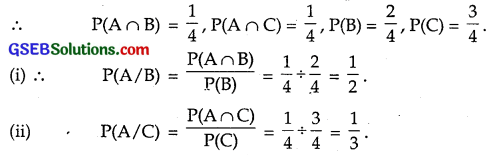Question 13.
An instructor has a test bank consisting of 300 easy True/False questions, 200 difficult True/False questions, 500 easy multiple choice questions and 400 difficult multiple choice questions.
If a question is selected at random from the test bank, what is the probability that it will be an easy questions given that it is a multiple choice questions?
Solution:
The given data may be tabulated as follows:Let us denote E = Easy questions, D = Difficult questions, T = True/False questions and M = Multiple choice questions.
Number of easy multiple choice questions = 500.
Total number of questions = 1400.
P(E ∩ M) = Probability of selecting an easy and multiple choice question
= $$\frac{500}{1400}$$.
Total number of multiple choice questions
= 500 + 400 = 900
P(M) = Probability of selecting one multiple choice question
= $$\frac{900}{1400}$$
∴ P(E/M) = $$\frac{P(E∩M)}{P(M)}$$ = $$\frac{500}{1400}$$ ÷ $$\frac{900}{1400}$$ = $$\frac{5}{9}$$.

Question 14.
Given that two numbers appearing on throwing two dice are different. Find the probability of the event ‘the sum of the numbers on the dice is 4’.
Solution:
When the numbers appearing on throwing two dice are different.
⇒ The numbers are not the same
Total number of exhaustive cases = 36
Number of cases when doublets do not occur = 36 – 6 = 30
Cases when the sum is 4 are {(1, 3), (2, 2), (3, 1)}
Let A denotes the event when the sum of numbers on two dice is 4 and B is the event when the numbers appearing on the dice are different.
∴ A ∩ B = {(1, 3), (3, 1)}
So, P(A ∩ B) = $$\frac{2}{36}$$, P(B) = $$\frac{30}{36}$$.
∴ P(A/B) = $$\frac{P(A∩B)}{P(B)}$$ = $$\frac{2}{36}$$ ÷ $$\frac{3}{36}$$ = $$\frac{1}{15}$$.Question 15.
Consider the experiment of throwing a dice, ‘if a multiple of 3 comes up, throw the dice again and if any other number comes toss a coin’.
Find the conditional probability of the event ‘the coin shows tail’, given that at least one dice shows a 3.
Solution:
Let there be n throws in which a multiple of 3 occurs every time.
Probability of getting a multiple of 3 (i.e., 3 or 6) in one throw
= $$\frac{2}{6}$$ = $$\frac{1}{3}$$.
∴ Probability of getting a multiple of 3 in n throws = ($$\frac{1}{3}$$)n
Probability of getting a 6 in one throw = $$\frac{1}{6}$$
∴ Probability of getting a 6 in n throws = ($$\frac{1}{6}$$)n.
⇒ Probability of getting at least a 3 in n throws = ($$\frac{1}{3}$$)n – ($$\frac{1}{6}$$)n
Let a multiple of 3 does not occur in (n + 1)th throw.
∴ Probability of getting 1, 2, 4, 5 (not a multiple of 3) in
(n + 1)th throw = $$\frac{4}{6}$$ = $$\frac{2}{3}$$.
In the next throw, a coin is tossed and tail occurs.
∴ Probability of getting a tail = $$\frac{1}{2}$$
⇒ Probability of getting at least a 3 and a tail in the end inQuestion 16.
If P(A) = $$\frac{1}{2}$$, P(B) = 0, then P(A/B) is
(A) 0
(B) $$\frac{1}{2}$$
(C) not defined
(D) 1
Solution:
P(A) = $$\frac{1}{2}$$, P(B) = 0
P(A/B) = $$\frac{P(A∩B)}{P(B)}$$ = $$\frac{P(A∩B)}{0}$$ = Not defined.
∴ Part (C) is the correct answer.Question 17.
If A and B are events such that P(A/B) = P(B/A), then
(A) A ⊂ B
(B) B = A
(C) A ∩ B = ∅
(D) P(A) = P(B)
Solution:
P(A/B) = P(B/A)
⇒ $$\frac{P(A∩B)}{P(B)}$$ = $$\frac{P(A∩B)}{P(A)}$$
⇒ P(A) = P(B)
∴ Part (D) is the correct answer.